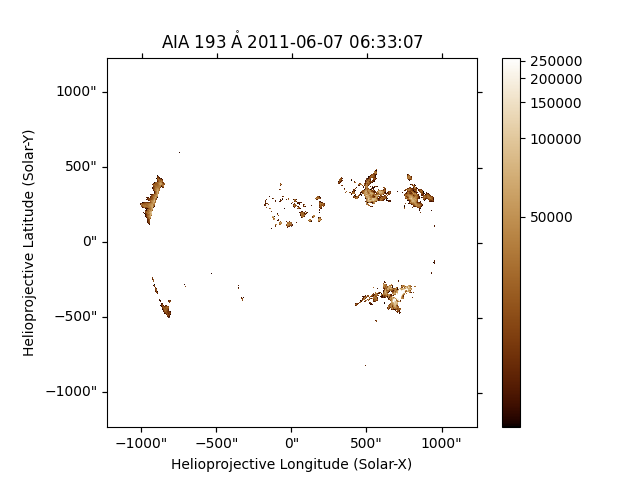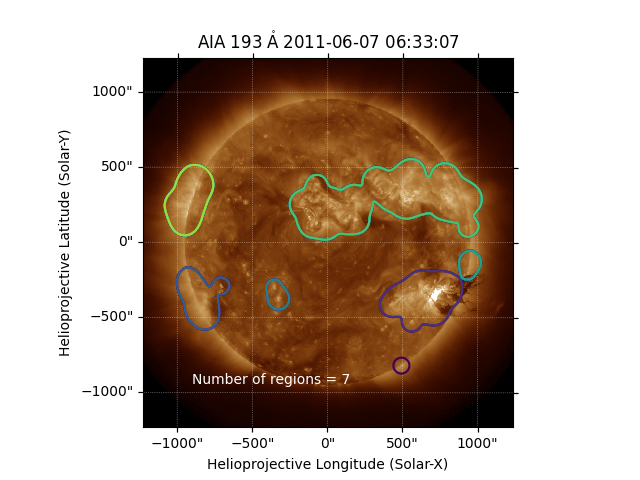# Manipulating Map image data - finding bright regions¶

This example shows how you can do basic image processing on SunPy map image data. In this example, we try to find the brightest regions in an AIA image. Then count the approximate number of regions of interest.

First, import the modules we will need:

from __future__ import print_function, division

from scipy import ndimage
import matplotlib.pyplot as plt

import sunpy.map
from sunpy.data.sample import AIA_193_IMAGE


Now, we create a SunPy Map object from an AIA FITS file.

aiamap = sunpy.map.Map(AIA_193_IMAGE)


Let’s plot the map. The aiamap normalizes the range using an asinh function. This show features in an image with high dynamic range more clearly.

plt.figure()
aiamap.plot()
plt.colorbar()
plt.show()Now we want to find the brightest regions in this image. We start by finding the maximum value in the image data.

data_max = aiamap.max()


Now we want to make a mask, which tells us which regions are bright. We choose the criterion that the data should be at least 5% of the maximum value. Pixels with intensity values greater than this are included in the mask, while all other pixels are excluded.

mask = aiamap.data < data_max * 0.05


Mask is a boolean array. It can be used to modify the original map object without modifying the data. Once this mask attribute is set, we can plot the image again.

aiamap.mask = mask
plt.figure()
aiamap.plot()
plt.colorbar()
plt.show()Only the brightest pixels remain in the image. However, these areas are artificially broken up into small regions. Estimating the number of significant hot regions will be difficult. We can solve this by applying some smoothing to the image data. Here we apply a 2D Gaussian smoothing function to the data.

data2 = ndimage.gaussian_filter(aiamap.data * ~mask, 16)


The issue with the filtering is that it create pixels where the values are small (<100), so when we go on later to label this array, we get one large region which encompasses the entire array. If you want to see, just remove this line.

data2[data2 < 100] = 0


Now we will make a second SunPy map with this smoothed data.

aiamap2 = sunpy.map.Map(data2, aiamap.meta)


The function label from the scipy.ndimage module, counts the number of contiguous regions in an image.

labels, n = ndimage.label(aiamap2.data)


Finally, we plot the smoothed bright image data, along with the estimate of the number of distinct regions. We can see that approximately 6 distinct hot regions are present above the 5% of the maximum level.

plt.figure()
aiamap2.plot()
plt.contour(labels)
plt.figtext(0.3, 0.2, 'Number of regions = {}'.format(n), color='white')
plt.show()Total running time of the script: ( 0 minutes 1.710 seconds)

Gallery generated by Sphinx-Gallery